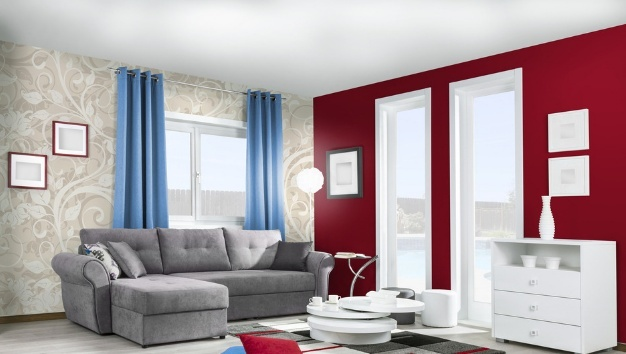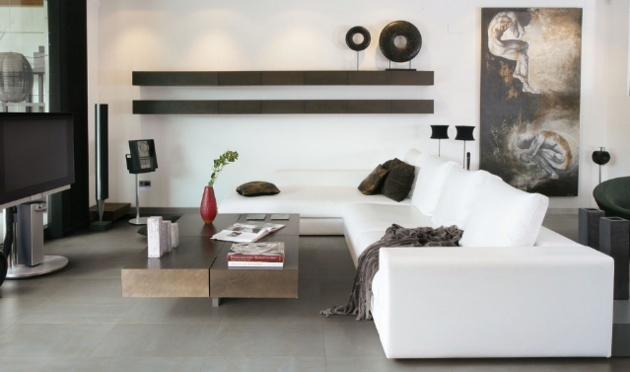|

# 元洲装饰套餐明细表 元洲服务承诺

众所周知，元洲装饰乃是家居装饰行业的领军者，京城百姓信赖的十大品牌之一。随着装饰企业竞争互仿，套餐装修也进入了激烈的竞争阶段。那么面对市场上各种家装套餐而言，我们该如何选择适合自己的家装套餐呢？今天，小编就给大家介绍下元洲装饰套餐，及元洲服务承诺，希望给您一个满意的定心丸！元洲装饰套餐明细表 元洲服务承诺

Part1：元洲装饰全包套餐装修明细清单

一、主卧室

轻钢龙骨石膏板平顶

轻钢龙骨石膏板直线造型顶

地面找平

墙、顶面基层处理

华润净味墙面漆

墙、顶面基层铲除

踢脚线（木质成品）

二、次卧

顶棚乳胶漆

墙面乳胶漆

门垛或门楣（1个）

强化复合地板

地板踢脚线

套装门

【玄关、餐厅、客厅及过廊】

顶棚乳胶漆

墙面乳胶漆

强化复合地板

地板踢脚线

电视墙壁挂处理

入户门门口

开关面板

【厨房】

贴墙砖

贴地砖

包立管

假梁

主材墙砖（高度2.6m）

主材地砖（满铺）

铝扣板吊顶（满铺）

吸顶灯一个

橱柜地柜 3米

橱柜上柜 1.2米

洗菜盆

烟机

炉具

【卫生间】

贴墙砖

贴地砖

包立管

假梁

防水

座便器

浴室柜

龙头及下水翻板

角阀

花洒

主材墙砖（高度2.6m）

主材地砖（满铺）

铝扣板吊顶（满铺）

吸顶灯 浴霸 三合 1

地漏

推拉门

【水电路改造】

配电箱移位安装

强电布线明装（2.5平方塑铜线）

强电布线明装（4平方塑铜线）

强电布线明装（6平方塑铜线）

弱电视频线（明装）

网线替代电话线（明装）

水电路开线槽（砖墙）

水电路开线槽（混凝土）

下线盒（含分线盒）

开槽布线后水泥或石膏抹平

弱电网线改造布线

下水管安装

冷、热水PPR管改造 （伟星纳米4分抗菌水管）

PP-R上水管与原有水管及其它管材连接转换接头安装

【其它】

材料搬运费 （按建筑面积计算）

材料运输费 （按建筑面积计算）

室内垃圾清运费 （按建筑面积计算）

成品维护费 （按建筑面积计算）元洲装饰套餐明细表 元洲服务承诺

Part2：元洲装饰服务承诺

1.先行赔付

元洲魔方家居馆对馆内所有商户的经营活动承担连带责任，凡元洲魔方家居馆售出产品，公开接受客户监督。当客户因商品或服务等原因产生投诉，最后难以达成协议不能解决的，经过事实认定后，客户可以要求元洲魔方家居馆"先行赔付"。

2.30天无理由退货

客户在元洲魔方家居馆与商户达成购物意向、并开具元洲魔方家居馆统一销售合同单据(需有元洲魔方家居馆财务章)及统一收款单据，支付定金之日起30天内，如对购买产品感到不满意、不合适、不喜欢(30天无理由退货细则内容中不在30天无理由退货范围之内的除外)，可至元洲魔方家居馆进行无理由退货，不需作出任何解释。

3.同城比价3倍退差

客户在元洲魔方家居馆购买的正常商品(非定制、样品)，如在7天之内发现同城同类型商场、同一品牌、同一型号/规格产品，价格低于元洲魔方家居馆，凭该商场有效购物凭证，我们将给予3倍退差。(不同城市/地区商场、不同品牌、不同型号/规格商品、个别商场特别优惠处理的商品，所出现的价差，不在退差范畴；另外，基于定制商品的不可比性，故定制商品不在退差范畴)。

4.售出产品负全责

凡在元洲魔方家居馆内购买商品，并持有元洲魔方家居馆统一销售合同单据(需加盖元洲魔方家居馆售后服务章)及统一收款单据，或者有确实证据证明为在元洲魔方家居馆内购买的商品，客户因产品质量问题(产品质量问题认定以国家相关法律法规为依据)而产生疑议与投诉，在国家法律规定的三包期限内，元洲魔方家居馆商场负责全程处理，并承担相应责任。

5.倡导绿色环保政策

元洲魔方家居馆致力于倡导绿色环保，做行业领跑者，实行商户准入资质审查制度，力争达到商场产品绿色环保，从健康、美观、实用角度出发，努力为客户营造温馨、和谐家园。

6.送货安装准时达

在元洲魔方家居馆，运费、安装费从未被加进您购买家具的售价中。只要您需要，我们为您提供送货、安装服务(具体送货范围及标准以定单签订为准)。在元洲魔方家居馆经营的所有家具建材商家都必须按合同约定时间完成送货和安装任务，否则就要承担违约责任，即：每延迟一天按已付货款的3‰向客户支付违约金；超过30天，客户有权终止合同。

7.家居顾问全程导购

如果您有需要，可以至魔方家居馆服务台获得帮助，我们将安排专业的家居顾问，根据您的生活需要，为您量身推荐家居采购方案，为您省时省心。

8.设计师免费咨询

元洲魔方家居馆提供专业的家装设计、家具设计咨询服务。

9.免费停车

元洲魔方家居馆为驾车前往家居馆的客户提供充足的停车位，并不收取任何停车费用。元洲装饰套餐明细表 元洲服务承诺

`声明：本文由入驻焦点开放平台的作者撰写，除焦点官方账号外，观点仅代表作者本人，不代表焦点立场错误信息举报电话： 400-099-0099，邮箱：jubao@vip.sohu.com，或点此进行意见反馈，或点此进行举报投诉。`A B C D E F G H J K L M N P Q R S T W X Y Z
A - B - C - D - E
• A
• 鞍山
• 安庆
• 安阳
• 安顺
• 安康
• 澳门
• B
• 北京
• 保定
• 包头
• 巴彦淖尔
• 本溪
• 蚌埠
• 亳州
• 滨州
• 北海
• 百色
• 巴中
• 毕节
• 保山
• 宝鸡
• 白银
• 巴州
• C
• 承德
• 沧州
• 长治
• 赤峰
• 朝阳
• 长春
• 常州
• 滁州
• 池州
• 长沙
• 常德
• 郴州
• 潮州
• 崇左
• 重庆
• 成都
• 楚雄
• 昌都
• 慈溪
• 常熟
• D
• 大同
• 大连
• 丹东
• 大庆
• 东营
• 德州
• 东莞
• 德阳
• 达州
• 大理
• 德宏
• 定西
• 儋州
• 东平
• E
• 鄂尔多斯
• 鄂州
• 恩施
F - G - H - I - J
• F
• 抚顺
• 阜新
• 阜阳
• 福州
• 抚州
• 佛山
• 防城港
• G
• 赣州
• 广州
• 桂林
• 贵港
• 广元
• 广安
• 贵阳
• 固原
• H
• 邯郸
• 衡水
• 呼和浩特
• 呼伦贝尔
• 葫芦岛
• 哈尔滨
• 黑河
• 淮安
• 杭州
• 湖州
• 合肥
• 淮南
• 淮北
• 黄山
• 菏泽
• 鹤壁
• 黄石
• 黄冈
• 衡阳
• 怀化
• 惠州
• 河源
• 贺州
• 河池
• 海口
• 红河
• 汉中
• 海东
• I
• J
• 晋中
• 锦州
• 吉林
• 鸡西
• 佳木斯
• 嘉兴
• 金华
• 景德镇
• 九江
• 吉安
• 济南
• 济宁
• 焦作
• 荆门
• 荆州
• 江门
• 揭阳
• 金昌
• 酒泉
• 嘉峪关
K - L - M - N - P
• K
• 开封
• 昆明
• 昆山
• L
• 廊坊
• 临汾
• 辽阳
• 连云港
• 丽水
• 六安
• 龙岩
• 莱芜
• 临沂
• 聊城
• 洛阳
• 漯河
• 娄底
• 柳州
• 来宾
• 泸州
• 乐山
• 六盘水
• 丽江
• 临沧
• 拉萨
• 林芝
• 兰州
• 陇南
• M
• 牡丹江
• 马鞍山
• 茂名
• 梅州
• 绵阳
• 眉山
• N
• 南京
• 南通
• 宁波
• 南平
• 宁德
• 南昌
• 南阳
• 南宁
• 内江
• 南充
• P
• 盘锦
• 莆田
• 平顶山
• 濮阳
• 攀枝花
• 普洱
• 平凉
Q - R - S - T - W
• Q
• 秦皇岛
• 齐齐哈尔
• 衢州
• 泉州
• 青岛
• 清远
• 钦州
• 黔南
• 曲靖
• 庆阳
• R
• 日照
• 日喀则
• S
• 石家庄
• 沈阳
• 双鸭山
• 绥化
• 上海
• 苏州
• 宿迁
• 绍兴
• 宿州
• 三明
• 上饶
• 三门峡
• 商丘
• 十堰
• 随州
• 邵阳
• 韶关
• 深圳
• 汕头
• 汕尾
• 三亚
• 三沙
• 遂宁
• 山南
• 商洛
• 石嘴山
• T
• 天津
• 唐山
• 太原
• 通辽
• 铁岭
• 泰州
• 台州
• 铜陵
• 泰安
• 铜仁
• 铜川
• 天水
• 天门
• W
• 乌海
• 乌兰察布
• 无锡
• 温州
• 芜湖
• 潍坊
• 威海
• 武汉
• 梧州
• 渭南
• 武威
• 吴忠
• 乌鲁木齐
X - Y - Z
• X
• 邢台
• 徐州
• 宣城
• 厦门
• 新乡
• 许昌
• 信阳
• 襄阳
• 孝感
• 咸宁
• 湘潭
• 湘西
• 西双版纳
• 西安
• 咸阳
• 西宁
• 仙桃
• 西昌
• Y
• 运城
• 营口
• 盐城
• 扬州
• 鹰潭
• 宜春
• 烟台
• 宜昌
• 岳阳
• 益阳
• 永州
• 阳江
• 云浮
• 玉林
• 宜宾
• 雅安
• 玉溪
• 延安
• 榆林
• 银川
• Z
• 张家口
• 镇江
• 舟山
• 漳州
• 淄博
• 枣庄
• 郑州
• 周口
• 驻马店
• 株洲
• 张家界
• 珠海
• 湛江
• 肇庆
• 中山
• 自贡
• 资阳
• 遵义
• 昭通
• 张掖
• 中卫

1室1厅1厨1卫1阳台

1
2
3
4
5

0
1
2

1

1

0
1
2
3报名成功，资料已提交审核A B C D E F G H J K L M N P Q R S T W X Y Z
A - B - C - D - E
• A
• 鞍山
• 安庆
• 安阳
• 安顺
• 安康
• 澳门
• B
• 北京
• 保定
• 包头
• 巴彦淖尔
• 本溪
• 蚌埠
• 亳州
• 滨州
• 北海
• 百色
• 巴中
• 毕节
• 保山
• 宝鸡
• 白银
• 巴州
• C
• 承德
• 沧州
• 长治
• 赤峰
• 朝阳
• 长春
• 常州
• 滁州
• 池州
• 长沙
• 常德
• 郴州
• 潮州
• 崇左
• 重庆
• 成都
• 楚雄
• 昌都
• 慈溪
• 常熟
• D
• 大同
• 大连
• 丹东
• 大庆
• 东营
• 德州
• 东莞
• 德阳
• 达州
• 大理
• 德宏
• 定西
• 儋州
• 东平
• E
• 鄂尔多斯
• 鄂州
• 恩施
F - G - H - I - J
• F
• 抚顺
• 阜新
• 阜阳
• 福州
• 抚州
• 佛山
• 防城港
• G
• 赣州
• 广州
• 桂林
• 贵港
• 广元
• 广安
• 贵阳
• 固原
• H
• 邯郸
• 衡水
• 呼和浩特
• 呼伦贝尔
• 葫芦岛
• 哈尔滨
• 黑河
• 淮安
• 杭州
• 湖州
• 合肥
• 淮南
• 淮北
• 黄山
• 菏泽
• 鹤壁
• 黄石
• 黄冈
• 衡阳
• 怀化
• 惠州
• 河源
• 贺州
• 河池
• 海口
• 红河
• 汉中
• 海东
• I
• J
• 晋中
• 锦州
• 吉林
• 鸡西
• 佳木斯
• 嘉兴
• 金华
• 景德镇
• 九江
• 吉安
• 济南
• 济宁
• 焦作
• 荆门
• 荆州
• 江门
• 揭阳
• 金昌
• 酒泉
• 嘉峪关
K - L - M - N - P
• K
• 开封
• 昆明
• 昆山
• L
• 廊坊
• 临汾
• 辽阳
• 连云港
• 丽水
• 六安
• 龙岩
• 莱芜
• 临沂
• 聊城
• 洛阳
• 漯河
• 娄底
• 柳州
• 来宾
• 泸州
• 乐山
• 六盘水
• 丽江
• 临沧
• 拉萨
• 林芝
• 兰州
• 陇南
• M
• 牡丹江
• 马鞍山
• 茂名
• 梅州
• 绵阳
• 眉山
• N
• 南京
• 南通
• 宁波
• 南平
• 宁德
• 南昌
• 南阳
• 南宁
• 内江
• 南充
• P
• 盘锦
• 莆田
• 平顶山
• 濮阳
• 攀枝花
• 普洱
• 平凉
Q - R - S - T - W
• Q
• 秦皇岛
• 齐齐哈尔
• 衢州
• 泉州
• 青岛
• 清远
• 钦州
• 黔南
• 曲靖
• 庆阳
• R
• 日照
• 日喀则
• S
• 石家庄
• 沈阳
• 双鸭山
• 绥化
• 上海
• 苏州
• 宿迁
• 绍兴
• 宿州
• 三明
• 上饶
• 三门峡
• 商丘
• 十堰
• 随州
• 邵阳
• 韶关
• 深圳
• 汕头
• 汕尾
• 三亚
• 三沙
• 遂宁
• 山南
• 商洛
• 石嘴山
• T
• 天津
• 唐山
• 太原
• 通辽
• 铁岭
• 泰州
• 台州
• 铜陵
• 泰安
• 铜仁
• 铜川
• 天水
• 天门
• W
• 乌海
• 乌兰察布
• 无锡
• 温州
• 芜湖
• 潍坊
• 威海
• 武汉
• 梧州
• 渭南
• 武威
• 吴忠
• 乌鲁木齐
X - Y - Z
• X
• 邢台
• 徐州
• 宣城
• 厦门
• 新乡
• 许昌
• 信阳
• 襄阳
• 孝感
• 咸宁
• 湘潭
• 湘西
• 西双版纳
• 西安
• 咸阳
• 西宁
• 仙桃
• 西昌
• Y
• 运城
• 营口
• 盐城
• 扬州
• 鹰潭
• 宜春
• 烟台
• 宜昌
• 岳阳
• 益阳
• 永州
• 阳江
• 云浮
• 玉林
• 宜宾
• 雅安
• 玉溪
• 延安
• 榆林
• 银川
• Z
• 张家口
• 镇江
• 舟山
• 漳州
• 淄博
• 枣庄
• 郑州
• 周口
• 驻马店
• 株洲
• 张家界
• 珠海
• 湛江
• 肇庆
• 中山
• 自贡
• 资阳
• 遵义
• 昭通
• 张掖
• 中卫• 手机• 分享
• 设计
免费设计
• 计算器
装修计算器
• 入驻
合作入驻
• 联系
联系我们
• 置顶
返回顶部